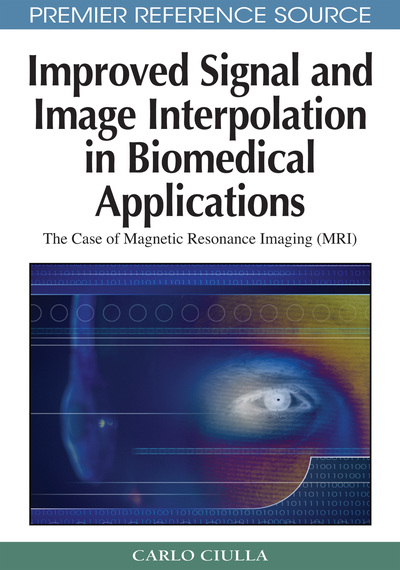# The Results of the Sub-Pixel Efficacy Region Based Trivariate Linear Interpolation Function

Carlo Ciulla (Lane College, USA)
DOI: 10.4018/978-1-60566-202-2.ch011
Available
\$37.50
No Current Special Offers

## Abstract

This chapter presents results relevant to the evaluation of the performance of classic and SRE-based trivariate interpolation functions. The forthcoming text reports on the validation procedure employed to quantify the interpolation error of the two model functions along with the information on the resolution of the MRI database that was processed. The presentation of the results is both quantitative through the plots of the RSME Ratio and quantitative through the presentation of the spectral power evolutions of functional MRI data. As anticipated in Chapter I the spectral power evolutions were obtained processing the fMRI volume with the misplacement X = 0.49, Y = 0.49, Z = 0.49 employing the two interpolation paradigms (classic and SRE-based). Particularly, the RSME Ratio quantified the relativity of performance between the two types of trivariate linear interpolation functions while processing T1-weigthed, T2-weighted and functional MRI. The spectral power evolutions quantified for the functional MRI the differences in frequency spectral content between the two classes of interpolators. Interesting to note that the spectral power evolutions clearly show in this chapter their capability to reveal differences, between the images obtained through the two different interpolators, which otherwise would not be observed in the image space. Such differences are somehow hidden in the k-space (Fourier domain).
Chapter Preview
Top

## Validation Procedure

At the aim to show the error improvement obtainable through the methodological approach of the unifying theory the same type of motion correction paradigm employed for the bivariate linear is presented in this chapter for the trivariate linear functions. An original three dimensional image is shifted. Original means prior to any processing. It is then motion corrected of the initial shift and interpolated with the classic trivariate linear function. This procedure is called processing with classic interpolation as the reader may recall from Chapter VIII of the book. The root-mean-square-error (RMSE) is calculated between original and processed images. Concurrently, the original image is processed with the SRE-based trivariate linear interpolation function (that means that the image is calculated at the novel re-sampling locations).

By doing so, the RMSE Ratio calculates a measure of the relativity of performance between classic and SRE-based trivariate linear interpolation functions. The performance is obviously measured on the basis of the interpolation error and so the RMSE Ratio allows elucidating which one of the two interpolation functions delivers best approximation.

Real Magnetic Resonance Imaging data were employed at the aim to study the interpolation error improvement determined through the SRE-based trivariate linear interpolation function and also to study the spectral characteristics of the re-sampled images as described in the following of this chapter. The data set is the same used for the validation of the SRE-based bivariate linear interpolation function and for convenience of the reader it is hereto recalled.

• T1 MRI (T1-MRI-230 and T1-MRI-450). Matrix resolution: 176 x 208 with 1.00 x 1.00 mm of pixel size, with 75 slices and an inter-slice resolution of 1.00 mm.

• T2 MRI. Matrix resolution: 177 x 182 with 1.00 x 1.00 mm of pixel size, with 19 slices and an inter-slice resolution of 1.00 mm.

• Functional MRI (fMRI-TS1). Matrix resolution: 128 x 128 with 1.72 x 1.72 mm of pixel size, with 20 slices and an inter-slice resolution of 1.72 mm.

• Functional MRI (fMRI-snffM00587). Matrix resolution: 53 x 63 with 3.00 x 3.00 mm of pixel size, with 30 slices and an inter-slice resolution of 3.00 mm.

Top

## Analysis Of The Rmse Ratio Obtained With Mri Data

The plot of the RMSE Ratio versus the concurrent misplacement along X, Y and Z directions is shown for T1-MRI-230, T1-MRI-450, T2-MRI, fMRI-TS1 and fMRI-snffM00587 in figures 1, 2, 3, 4, and 5 respectively. Each figure plots the RMSE ratio that is relevant to the entire MRI volume. The portion of the curves below zero shows that the SRE-based trivariate interpolation function outperforms the classic trivariate interpolation function. The portion of the curves above zero shows the opposite. For the four MRI data sets the range of misplacement was: [(0, 0, 0), (0.99, 0.99, 0.99)] at steps of (0.01, 0.01, 0.01).

## Complete Chapter List

Search this Book:
Reset